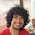### Sweet Heart Mix Tape

Source: Placement test of one of the Algorithmic Trading Firms coming to the campus. Also in UC Berkeley, Spring 2001 Final Exam for CS170 (Efficient Algorithms and Intractable Problems)

Problem: Pratik is organizing a mix tape for his sweetheart, Pratiksha. The tape will have her top N songs of all time. Pratik was going to determine the order of these songs on his own, but then Pratiksha found out about his little project. Being an obnoxiously demanding woman, she has now given Pratik a price function f which takes a pair of songs [si,sj] as input, and returns a real number that quantifies exactly how good song sj sounds after song si, in her opinion. (Note that f([si,sj]) may not be equal to f([sj,si]).)

Write an O(n^2*2^n) algorithm for Pratik that will determine a song order which maximizes the total transition goodness of the mix tape. (If the maximum is not achieved, Pratik will be dumped. :()

Solution:
Posted by Nikhil Garg (Sophomore, CSE, IITD), Rajendran Thirupugalsamy (Research Assistant, Stony Brook University) and "Anonymous" in comments!!

1.Consider the subproblem of finding the best schedule when we have a subset say S of songs available to play.

Denote by best(i,S) as best cost of arranging songs of set S with ith song as the first element.
Our final aim is max over i ( best (i,U) )
where U is the universal set of songs.

Now we can easily compute best(i,S) in terms of smaller such values :

if i does not belong to S
best(i,S)= -INF
else
best(i,S)= max over j ( best(j,S-{i} )+cost(i,j)

with appropriate base cases .

We have n*2^n subproblem to solve & each one requires O(n) time. Hence the solution.

2.You are right Nikhil.

Another similar problem is finding n*n*2^n solution for Travelling Salesman Problem using dynamic programming.

Infact, we can model this problem as a directed graph wherein, each song creates a vertex and the price value makes the distance. Now, what we need is solution to asymmetric TSP (except salesperson does not need to visit the starting city).

The dynamic programming solution for this follows the same way as Nikhil described and can be found here.

3.This is travelling salesman problem. you would need to add an additional start vertex whose distance to & from all given vertices is 0. TSP can be done O((n^2)*(2^n)) using dynamic programming.

4.Thanx Nikhil, Rajendran and "inexorable" for the solution

I too solved it in the same way. But is it really the travelling salesman problem? Not returning to the first node makes a difference. Can someone prove that this problem is indeed as hard as TSP.

5.isnt this a pretty normal-ish problem? i think we discussed this at work once, and i got it in a while...

6.@Ramdas.. finding the solution with dynammic programming is easy.. (The solution by Nikhil)

The point where I am not clear is that is this problem at least as hard as TSP? Can one prove some relation between this problem and TSP?

7.Yes. Traveling Salesman Path Problem (not returning to first node) is also in NP. We can reduce it via regular TSP.

First, model the TSPP problem as finding a path starting at a given vertex(s), visiting all vertices and ending at given vertex(t). Our Sweet-heart-mix-tape problem can use multiple calls to TSPP involving all combinations of s,t vertices to find the best solution.

Now we will prove TSPP is NP via a reduction from TSP. Given a TSP problem instance convert to TSPP instance as follows. Take a random single vertex(v) and split it into two vertices(a,b) and distance a->b = b->a = 0. Now finding the TSPP involving source as 'a' and dest as 'b', will give solution for TSP.

So TSPP is equally hard as TSP. As TSP in NP, TSPP is also in NP.

I also think the asymmetric price function (price(si,sj) may not be same as price(sj,si)) in our problem does not play much role.

8.Elegant proof. Thanx. You proved that the problem is atleast as hard as travelling salesman problem.

Thanx!